EXPERIMENT NO.12

End fire linear array with uniform spacing and amplitude

Practical Session Plan

 Time ( min) Content Learning Aid / Methodology Faculty Approach Typical Student Activity Skill / Competency Developed 10 Relevance and significance of experiment Chalk & Talk , Presentation Introduces, Facilitates, Monitors Listens, Participates, Discusses Knowledge, Communication, intrapersonal 20 Explanation of experiment Chalk & Talk , Presentation Introduces, Facilitates, Explains Listens Knowledge, Communication, intrapersonal, Application 20 Calculations N/A Explains, Monitors Participates, Discusses Knowledge, Communication, Intrapersonal, Application 60 Simulation Demonstration Explains, Monitors Participates, Discusses Debate, comprehension, Hands on experiment 10 Results and conclusions Keywords Lists, Facilitates Listens, Participates, Discusses Knowledge, Communication, intrapersonal, Comprehension

Title: End fire linear array with uniform spacing and amplitude

Aim:

Â· To sketch the radiation pattern of End fire Array with uniform spacing & amplitude

Â· To compute performance parameters

Objectives: Model the End fire Array to analyze the performance parameters

Apparatus: PC, EM Simulation software

Theory:

The end fire array is nothing but broadside array except that individual elements are fed in, out of phase. Thus in the end fire array, a number of identical antennas are spaced equally along a line and individual elements are fed with currents of equal magnitude but their phases varies progressively along the line in such a way as to make the entire arrangement substantially unidirectional. In other words, individual elements are exited in such a manner that a progressive phase difference b/w adjacent elements becomes equal to the spacing b/w the elements.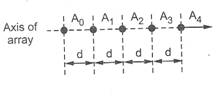Fig. 1 a Top View Fig.1b Front View

End Fire Array

End fire array defined as: the arrangement in which the principal direction of radiation coincides with the direction of the array axis.

It may be noted that end fire array may be bidirectional also.

Array of n elements with equal spacing and currents equal in Magnitude but with progressive phase shift:

Consider n no. Of identical radiators supplied with equal current which are not in phase, as shown in figure. Assume that there is a progressive phase lag of Î²d radians in each radiator.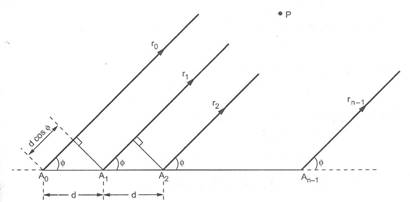Fig. 1 End Fire Array

Consider that the current supplied to first element A0 be I 0. Then the current supplied to A1 is given by,

I1= I0*e-jÎ²d

Similarly the current supplied to A2 is given by,

I2 = I1*I0*e-jÎ²d = [I 0*e-jÎ²d] e-jÎ²d= I0*e-2jÎ²d

Thus the current suppliedto last element is given by,

In-1 = I0*e-j (n-1)Î²d

The electric field produced at point P due to A0 is given by....... (1)

The electric field produced at point P due to A1 is given by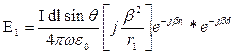But r1 = r0 â€“ d cosÎ¦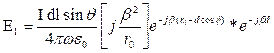E1 = E0*e jÎ²d(cosÎ¦-1) .........(2)

Let Î¨= Î²d(cosÎ¦-1)E1 = E0 e jÎ¨ ......... (3)

The electric field produced at point P due to A2 is given by

E2 = E0 e j2Î¨ ......... (4)

Similarly, the electric field produced at point P due to An-1 is given by,

En-1 = E0 e j(n-1)Î¨ ......... (5)

Thus the resultant field at point p is given by,

ET = E0+E1+E2+.......+E n-1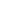ET = E0+E0 e jÎ¨+ E0 e j2Î¨ ..........+ E0 e j(n-1)Î¨ET = E0 [1+ e jÎ¨+ e j2Î¨ ..........+ e j (n-1) Î¨]

Using the concept of exponential series, we can write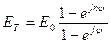Consider only magnitude, we getIn end fire Array the maximum radiation can be obtained along the axis of uniform end fire array if the progressive phase shift Î± between the elements is given by,= -Î²d For maximum in Î¦ = 0Â° direction

= +Î²d For maximum in Î¦ =180Â° direction

Procedure:

1. Calculate wavelength of dipole using the formula Î» = c/f, where c= 3*10 8& f is the frequency.

1. Initially open the notepad and write down the command given below

CM (Comment card)

CE (Comment enable)

EN (End)

Save the file with the extension .nec command

1. Close the notepad.
2. Click on the 4nec2 software shortcut icon present on desktop and then click on file option chooses open our saved notepad file.
3. Press control +F4 then window(filename.nec.txt) is opened, OR
4. Click on setting option and choose NEC editor (new) option
5. Click on edit NEC input file (Red colour book icon )
6. Choose symbol option and after choosing custom factor option if we want to do the calculation in meters then take 0.001 value (because 1m=1000mm)
7. Then select save and then click on structure is resized Ok.
8. Then choose geometry option. In type option we are making wire antennas so choose type is wire. Next option is tag which is a voltage source so it should keep as 1. Next is number of segments here we can choose any odd no. E.g. 3, 5, 7, 9, 11, 13........ In X, Y, Z we can write the length of our dipole as here our dipole is on Y axis so we given lambda/2 value on Y axis. Next is the radius of dipole i.e. thickness of conductor.
9. Then click on source/load option and specify voltage.
10. Then click on Frequency/Ground, and then specify the frequency and ground. Select frequency as given frequency, Environment= free space.
11. The next step is calculation of radiation pattern for that click on green book icon on main window for generating output select far field pattern option.
12. If we want to see 3D radiation pattern of antenna then click on 3D option on main window.
13. Take the print out for Geometry, Radiation pattern in 2D and 3D.

Design:

Use 4nec2 software to implement a End-fire array and its polar pattern for n = 4, d = 0.5Î»,

Î± = 180Â° and f = 300Mhz

f = 300Mhz

Î» = c/f

Î» = 3*108 / 300*106 = 1m.

d= spacing between the adjacent elementd = 0.5 Î» = 0.5 *1m = 0.5m

Simulation Input: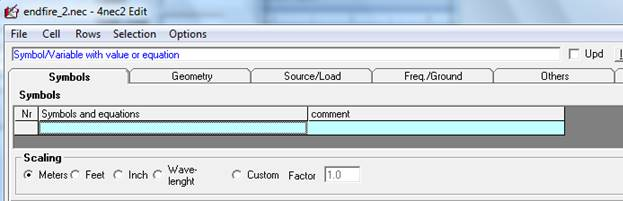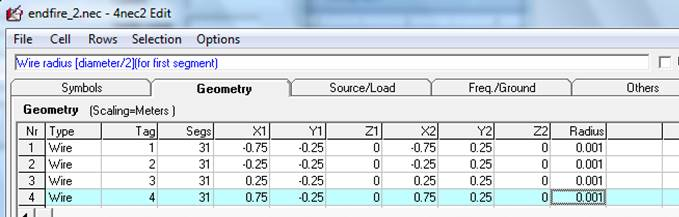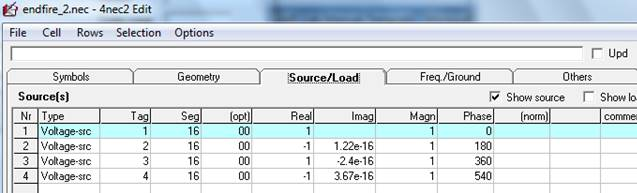Results: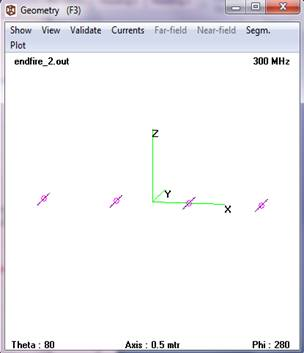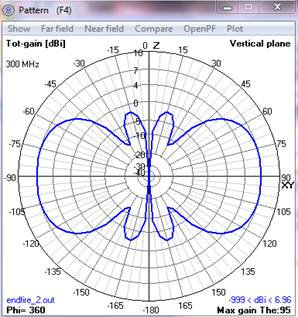Conclusion:

In Endfire array the maximum radiation is parallel to axis of antenna array i.e. at either 0o or 180o and beamwidth can be decreased by increasing number of elements.

Upon completion of experiment students will be able to:

1. Model the Endfire Array.

2. Analyze the performance parameters

Oral Question Bank

Theory Question Bank

 Q. No Description 1 What is an â€œarray factorâ€? 2 What are the types of antenna arrays? 3 Define the length of an array 4 What are the applications of arrays? 5 What is the direction of maximum radiation is maximum only in one direction that is in the direction of array axis. 6 Define antenna array. 7 What is an array and mention the various forms of antenna arrays? 8 What is array factor or space factor? 9 Write the principle of pattern multiplication? 10 Define HPBW? 11 Define FBR? 12 Define BWFN? 13 An array consisting a no. of equidistant dipoles of equal size, fed with the same currents and phase is called:(a) end fire array (b) back fire array (c) broad side array (d) binomial array 14 Define FSLBW? 15 What are the types of antenna arrays? 16 Define the length of an array? 17 What is array factor or space factor? 18 What are the applications of arrays? 19 What is a collinear array? 20 Which are the controlling parameters of the overall pattern of antenna array? 21 Why antenna arrays are required? 22 What is uniform linear array? 23 What is the effect of changing the number of element on the radiation pattern of an antenna array? 24 What is the effect of changing the spacing between adjacent elements on radiation pattern of an antenna array?